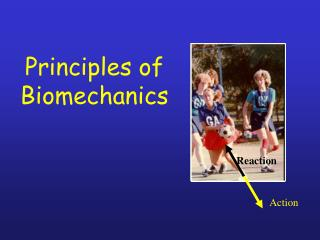DownloadDownload PresentationPrinciples of Biomechanics

# Principles of Biomechanics

Download Presentation## Principles of Biomechanics

- - - - - - - - - - - - - - - - - - - - - - - - - - - E N D - - - - - - - - - - - - - - - - - - - - - - - - - - -
##### Presentation Transcript

1. Principles of Biomechanics Reaction Action

2. Lesson Aim: • To critically examine the biomechanical principles which are important in Physical activity & Sport. • To be able to state why these principles are important to consider in terms of athletics.

3. Outline: • Newton’s Laws • Balance and Stability • Levers • Force Summation • Projectile Motion

4. Newton’s First Law of Motion • every body continues in it state of rest or motion in a straight line unless compelled to change that state by external forces exerted on it. (conservation of momentum) This external force will act to change the vertical motion, but not the horizontal

5. Newton’s Second Law of Motion • the rate of change of momentum (acceleration) of a object is proportional to the force causing it and the change takes place in the direction in which the force acts.

6. Newton’s Third Law of Motion • for every force that is exerted by one body on another, there is an equal and opposite force exerted by the second body on the first (action - reaction)

7. Balance

8. Balance C-o-M • The point about which the body’s mass is evenly distributed is referred to as the Centre of Mass (CoM) • This is also sometimes referred to as the Centre of Gravity, but this actually refers only to the vertical direction.

9. Balance C-o-M • The location of the CoM depends on the location of the body segments: • Raise your arms above your head and your CoM rises within your body • Lift one arm and one leg out to the side, and the CoM will move towards that side

10. Balance C-o-M • Understand the effect of positioning/moving limb segments while in the air… • if both of these people are the same height, have the same build and jump the same height, which one is going to hit the volleyball at the greatest height? Why?

11. Balance Base of support • The area that a body is in contact with a solid surface • Base of support can be between to or more points.

12. Balance unbalanced • A body becomes unbalanced when the centre of mass moves outside the base of support. • sometimes it is more valuable in athletics to be off balance, when why?

13. LEVERS

14. 3rd 1st 2nd F F R (BW) F R R Levers • “a bar, hinged/pivoted at one point, to which forces are applied at two other points” • Fulcrum/axis • Weight/resistance • Force

15. F R F R F R F R F R Levers – functional aspects

16. M R 3kg 4kg Most levers in the body are 3rd class • …… we’re built for speed, not force!

17. Force Summation

18. Inter-segment Sequencing • when the second segment reaches its maximum angular velocity (zero acceleration) during the midrange of its motion, it too decelerates and transfers angular momentum to the next adjacent distal segment in the chain. • such a sequence occurs, link by link, from large to small, from base to end (proximal to distal) until the end of the chain is reached.

19. H FA UA VS Force Summation W E rUA S

20. angular velocity time Force Summation

21. Projectile Motion

22. What are the characteristics of a high jumper as a projectile? What effect does varying the height, angle or speed of release have on the distance achieved? What effect does air resistance have on flight? Projectiles We typically look at Release height Release angle Release speed to evaluate projectile motion

23. Release angle: there is an optimum angle depending on the release height: Release ht = landing ht:  = 45 Release ht > landing ht:  < 45 Release ht < landing ht:  > 45 • Release speed: an increase in speed = increased range • Release height: increased height = increased range

24. Magnus Effect • if a ball spins in flight, it will drag some of the air close to the surface with it. This creates an area of high pressure and an area of low pressure on opposite sides of the ball • this pressure imbalance will make the ball curve in flight

25. Ignoring air resistance, the flight of a projectile will take a parabolic path • Horizontal velocity will be constant • Vertical velocity will change by -9.8 m/s/s